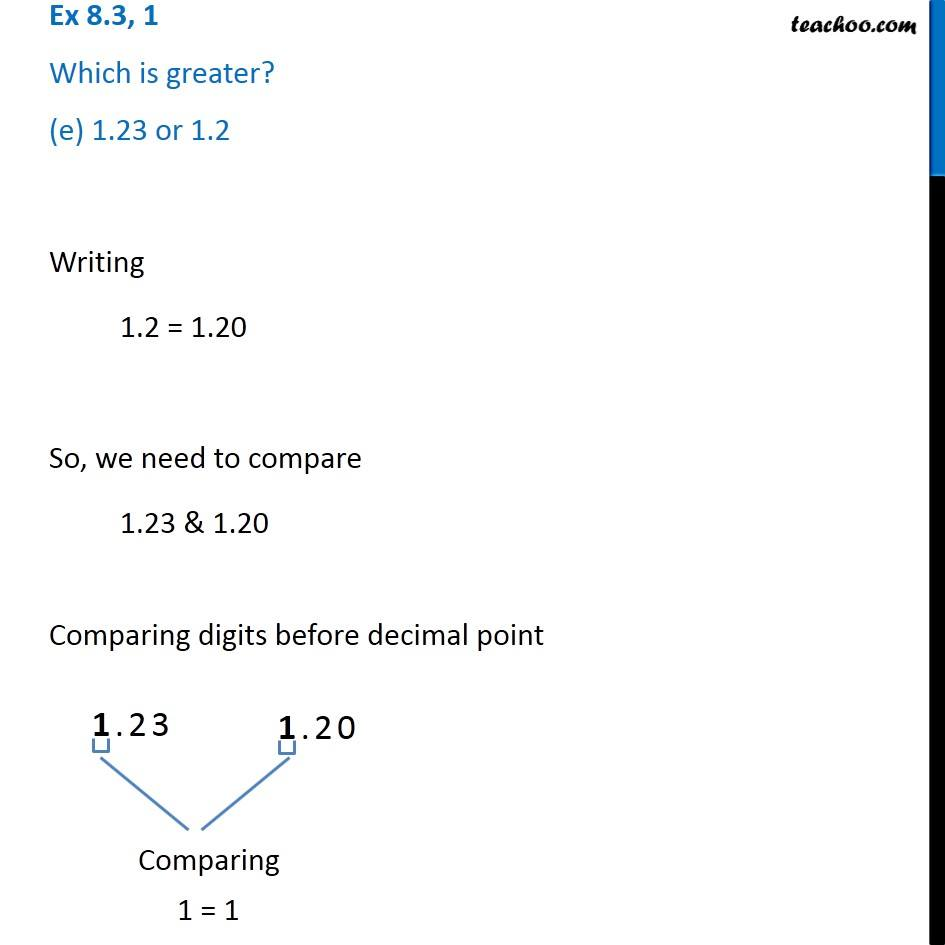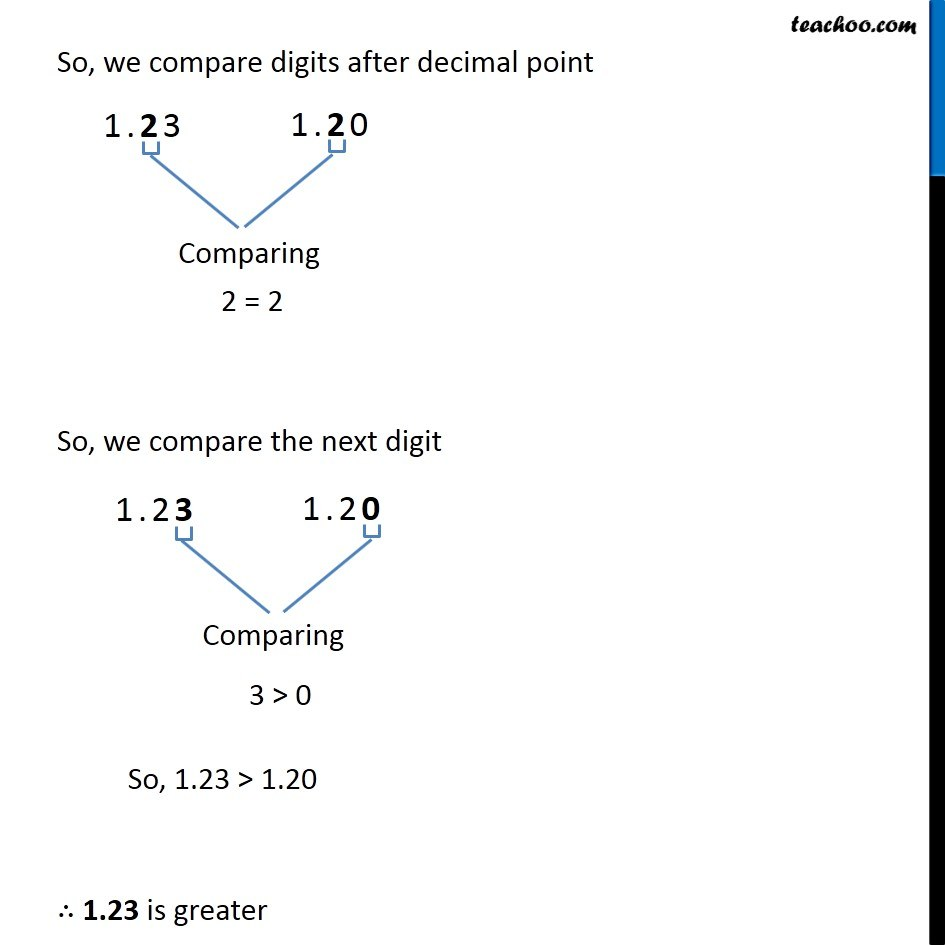1. Chapter 8 Class 6 Decimals
2. Concept wise
3. Comparing decimals

Transcript

Ex 8.3, 1 Which is greater? (e) 1.23 or 1.2 Writing 1.2 = 1.20 So, we need to compare 1.23 & 1.20 Comparing digits before decimal point 1 = 1 So, we compare digits after decimal point 2 = 2 So, we compare the next digit 3 > 0 So, 1.23 > 1.20 1.23 is greater

Comparing decimals